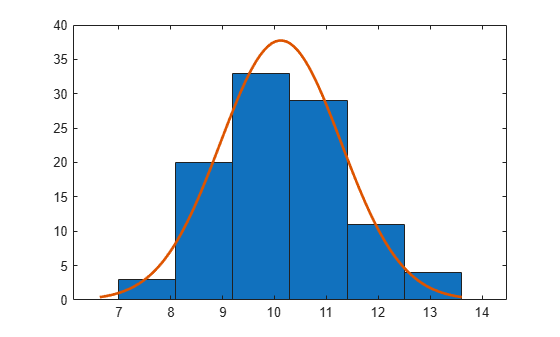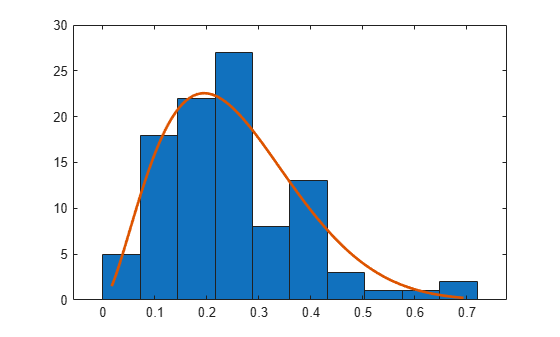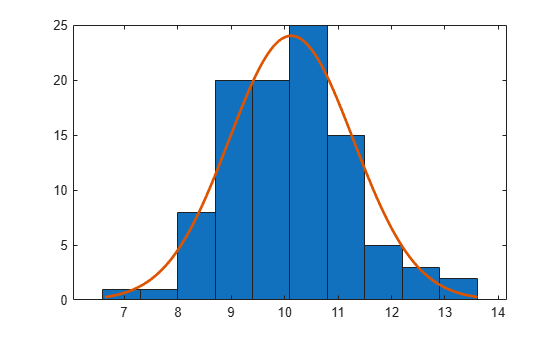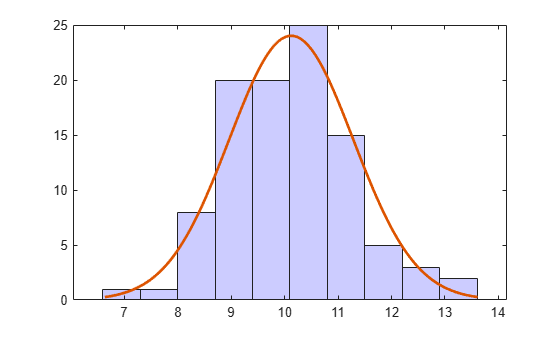# histfit

Histogram with a distribution fit

## Syntax

``histfit(data)``
``histfit(data,nbins)``
``histfit(data,nbins,dist)``
``histfit(ax,___)``
``h = histfit(___)``

## Description

example

````histfit(data)` plots a histogram of values in `data` using the number of bins equal to the square root of the number of elements in `data` and fits a normal density function.```

example

````histfit(data,nbins)` plots a histogram using `nbins` bins and fits a normal density function.```

example

````histfit(data,nbins,dist)` plots a histogram with `nbins` bins and fits a density function from the distribution specified by `dist`.```

example

````histfit(ax,___)` uses the plot axes specified by the `Axes` object `ax`. Specify `ax` as the first input argument followed by any of the input argument combinations in the previous syntaxes.```

example

````h = histfit(___)` returns a vector of handles `h`, where `h(1)` is the handle to the histogram and `h(2)` is the handle to the density curve.```

## Examples

collapse all

Generate a sample of size 100 from a normal distribution with mean 10 and variance 1.

```rng default; % For reproducibility r = normrnd(10,1,100,1);```

Construct a histogram with a normal distribution fit.

`histfit(r)``histfit` uses `fitdist` to fit a distribution to data. Use `fitdist` to obtain parameters used in fitting.

`pd = fitdist(r,'Normal')`
```pd = NormalDistribution Normal distribution mu = 10.1231 [9.89244, 10.3537] sigma = 1.1624 [1.02059, 1.35033] ```

The intervals next to the parameter estimates are the 95% confidence intervals for the distribution parameters.

Generate a sample of size 100 from a normal distribution with mean 10 and variance 1.

```rng default; % For reproducibility r = normrnd(10,1,100,1);```

Construct a histogram using six bins with a normal distribution fit.

`histfit(r,6)`Generate a sample of size 100 from a beta distribution with parameters (3,10).

```rng default; % For reproducibility b = betarnd(3,10,100,1);```

Construct a histogram using 10 bins with a beta distribution fit.

`histfit(b,10,'beta')`Generate a sample of size 100 from a beta distribution with parameters (3,10).

```rng default; % For reproducibility b = betarnd(3,10,[100,1]);```

Construct a histogram using 10 bins with a smoothing function fit.

`histfit(b,10,'kernel')`Generate a sample of size `100` from a normal distribution with mean `3` and variance `1`.

```rng('default') % For reproducibility r = normrnd(3,1,100,1);```

Create a figure with two subplots and return the `Axes` objects as `ax1` and `ax2`. Create a histogram with a normal distribution fit in each set of axes by referring to the corresponding `Axes` object. In the left subplot, plot a histogram with 10 bins. In the right subplot, plot a histogram with 5 bins. Add a title to each plot by passing the corresponding `Axes` object to the `title` function.

```ax1 = subplot(1,2,1); % Left subplot histfit(ax1,r,10,'normal') title(ax1,'Left Subplot') ax2 = subplot(1,2,2); % Right subplot histfit(ax2,r,5,'normal') title(ax2,'Right Subplot')```Generate a sample of size 100 from a normal distribution with mean 10 and variance 1.

```rng default % for reproducibility r = normrnd(10,1,100,1);```

Construct a histogram with a normal distribution fit.

`h = histfit(r,10,'normal')````h = 2x1 graphics array: Bar Line ```

Change the bar colors of the histogram.

`h(1).FaceColor = [.8 .8 1];`Change the color of the density curve.

`h(2).Color = [.2 .2 .2];`## Input Arguments

collapse all

Input data, specified as a vector.

Example: `data = [1.5 2.5 4.6 1.2 3.4]`

Example: `data = [1.5 2.5 4.6 1.2 3.4]'`

Data Types: `double` | `single`

Number of bins for the histogram, specified as a positive integer. Default value is the square root of the number of elements in `data`, rounded up. Use [ ] for the default number of bins when fitting a distribution.

Example: `y = histfit(x,8)`

Example: `y = histfit(x,10,'gamma')`

Example: `y = histfit(x,[ ],'weibull')`

Data Types: `double` | `single`

Distribution to fit to the histogram, specified as a character vector or string scalar. The following table shows the supported distributions.

`dist`Description
`'beta'`Beta
`'birnbaumsaunders'`Birnbaum-Saunders
`'burr'`Burr Type XII
`'exponential'`Exponential
`'extreme value'` or `'ev'`Extreme value
`'gamma'`Gamma
`'generalized extreme value'` or `'gev'`Generalized extreme value
`'generalized pareto'` or `'gp'`Generalized Pareto (threshold 0)
`'inversegaussian'`Inverse Gaussian
`'logistic'`Logistic
`'loglogistic'`Loglogistic
`'lognormal'`Lognormal
`'nakagami'`Nakagami
`'negative binomial'` or `'nbin'`Negative binomial
`'normal'`Normal
`'poisson'`Poisson
`'rayleigh'`Rayleigh
`'rician'`Rician
`'tlocationscale'`t location-scale
`'weibull'` or `'wbl'`Weibull
`'kernel'`Nonparametric kernel-smoothing distribution. The density is evaluated at 100 equally spaced points that cover the range of the data in `data`. It works best with continuously distributed samples.

Axes for the plot, specified as an `Axes` object. If you do not specify `ax`, then `histfit` creates the plot using the current axes. For more information on creating an `Axes` object, see `axes`.

## Output Arguments

collapse all

Handles for the plot, returned as a vector, where `h(1)` is the handle to the histogram, and `h(2)` is the handle to the density curve. `histfit` normalizes the density to match the total area under the curve with that of the histogram.

## Algorithms

`histfit` uses `fitdist` to fit a distribution to data. Use `fitdist` to obtain parameters used in fitting.

## Version History

Introduced before R2006a